# 图像处理中flip

## 图像处理总结概念

2018-05-08 13:29:41 dajiyi1998 阅读数 19487
• ###### 车道线检测相关理论知识点

通过本课程学习，初学者可以熟练使用opencv4 API完成各种常见的图像分割、识别任务。 高级学习者也一定能有新的体会和收获。 本课程所讲的例子代码来自于实际开发项目，有较高的实用性。

8人学习 刘山
免费试看

1.直方图：一幅图像由不同灰度值的像素组成，图像中灰度的分布情况是该图像的一个重要特征。图像的灰度直方图就描述了图像中灰度分布情况，能够很直观的展示出图像中各个灰度级所占的多少。图像的灰度直方图是灰度级的函数，描述的是图像中具有该灰度级的像素的个数：其中，横坐标是灰度级，纵坐标是该灰度级出现的频率。

rK是去见[0，G]内的第K级亮度，nk是灰度级为rK的像素数。

1.1直方图对比：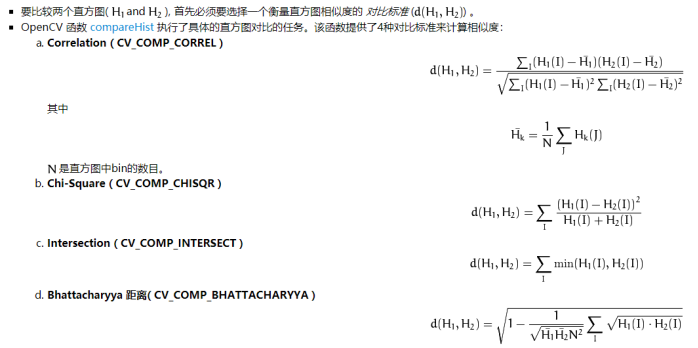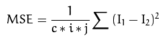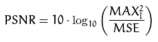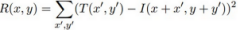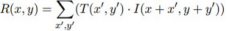1.2反向投影：一种记录给定图像中像素点如何适应直方图模型像素分布方式的一种方法，也就是说首先计算某一种特征的直方图模板，然后使用模板在去寻找图像中存在的该特征的方法。  作用：反向投影用于在输入图像（通常较大）中查找特定图像（通常较小或者仅1个像素，以下将其称为模板图像）最匹配的点或者区域，也就是定位模板图像出现在输入图像的位置。

1）从输入图像的左上角(0,0)开始，切割一块(0,0)至(10,10)的临时图像；

2）生成临时图像的直方图；

3）用临时图像的直方图和模板图像的直方图对比，对比结果记为c；

4）直方图对比结果c，就是结果图像(0,0)处的像素值；

5）切割输入图像从(0,1)至(10,11)的临时图像，对比直方图，并记录到结果图像；

6）重复（1）～（5）步直到输入图像的右下角。

1.3.直方图均衡化：如果一副图像的像素占有很多的灰度级而且分布均匀，那么这样的图像往往有高对比度和多变的灰度色调。直方图均衡化就是一种能仅靠输入图像直方图信息自动达到这种效果的变换函数。它的基本思想是对图像中像素个数多的灰度级进行展宽，而对图像中像素个数少的灰度进行压缩，从而扩展像元取值的动态范围，提高了对比度和灰度色调的变化，使图像更加清晰。直方图均衡化是图像处理领域中利用图像直方图对对比度进行调整的方法。这种方法通常用来增加许多图像的局部对比度，尤其是当图像的有用数据的对比度相当接近的时候。通过这种方法，亮度可以更好地在直方图上分布。这样就可以用于增强局部的对比度而不影响整体的对比度，直方图均衡化通过有效地扩展常用的亮度来实现这种功能。

(1)EQ(f)在0≤f≤L-1范围内是一个单值单增函数。这是为了保证增强处理没有打乱原始图像的灰度排列次序，原图各灰度级在变换后仍保持从黑到白(或从白到黑)的排列。

(2)对于0≤f≤L-1有0≤g≤L-1，这个条件保证了变换前后灰度值动态范围的一致性。

2.滤波：图像滤波，即在尽量保留图像细节特征的条件下对目标图像的噪声进行抑制，是图像预处理中不可缺少的操作，其处理效果的好坏将直接影响到后续图像处理和分析的有效性和可靠性。目的：1、消除图像中混入的噪声；2、为图像识别抽取出图像特。要求：1、不能损坏图像轮廓及边缘 ；2、图像视觉效果应当更好。

2.1.线性滤波器：在图像处理中，对邻域中的像素的计算为线性运算时，如利用窗口函数进行平滑加权求和的运算，或者某种卷积运算，都可以称为线性滤波。常见的线性滤波有：均值滤波、高斯滤波、盒子滤波、拉普拉斯滤波等等，通常线性滤波器之间只是模版系数不同。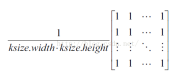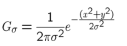2.2. 非线性滤波：非线性滤波利用原始图像跟模版之间的一种逻辑关系得到结果，如最值滤波器，中值滤波器。比较常用的有中值滤波器和双边滤波器。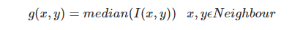w(x,y)为加权系数，取决于定义域核和值域核的乘积。

3边缘检测：Edge Detection 我们要找水平的边缘：需要注意的是，这里矩阵的元素和是0，所以滤波后的图像会很暗，只有边缘的地方是有亮度的。边缘是图像中灰度发生急剧变化的区域边界。图像灰度的变化情况可以用图像灰度分布的梯度来表示，数字图像中求导是利用差分近似微分来进行的，实际上常用空域微分算子通过卷积来完成。

1）图像滤波：传统边缘检测算法主要是基于图像强度的一阶和二阶导数，但导数的计算对噪声很敏感，因此必须使用滤波器来改善与噪声有关的边缘检测器的性能。需要指出的是，大多数滤波器在降低噪声的同时也造成了了边缘强度的损失，因此，在增强边缘和降低噪声之间需要一个折衷的选择。

2）图像增强：增强边缘的基础是确定图像各点邻域强度的变化值。增强算法可以将邻域(或局部)强度值有显著变化的点突显出来。边缘增强一般是通过计算梯度的幅值来完成的。

3）图像检测：在图像中有许多点的梯度幅值比较大，而这些点在特定的应用领域中并不都是边缘，所以应该用某种方法来确定哪些点是边缘点。最简单的边缘检测判断依据是梯度幅值。

4）图像定位：如果某一应用场合要求确定边缘位置，则边缘的位置可在子像素分辨率上来估计，边缘的方位也可以被估计出来。近20多年来提出了许多边缘检测算子。

1）Roberts算子：是一种斜向偏差分的梯度计算方法，梯度的大小代表边缘的强度，梯度的方向与边缘的走向垂直。Roberts操作实际上是求旋转45度两个方向上微分值的和。定位精度高，在水平和垂直方向的效果好，但对噪声敏感。两个卷积核Gx、Gy分别为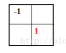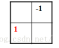2)Sobel算子：是一组方向算子，从不同的方向检测边缘。Sobel算子不是简单的求平均再差分，而是加强了中心像素上下左右4个方向像素的权值，运算结果是一副边缘图。Sobel算子通常对灰度渐变和噪声较多的图像处理的比较好。两个卷积核Gx、Gy分别为：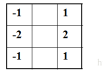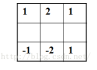3）Prewitt算子：是一种边缘样板算子，利用像素点上下左右邻点灰度差，在边缘处达到极值检测边缘，对噪声具有平滑的作用。由于边缘点像素的灰度值与其邻域点的灰度值显著不同，在实际应用中通常采用微分算子和模板匹配的方法检测图像的边缘。Prewitt算子不仅能检测边缘点，而且能抑制噪声的影响，因此对灰度和噪声较多的图像处理得比较好。两个卷积核Gx、Gy分别为：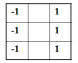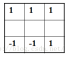1)  Laplacian算子：拉普拉斯算子是一种常用的二阶导数算子。实际中可根据二阶导数算子过零点的性质来确定边缘的位置。对于一个连续函数f(x,y),它在位置(x,y)的拉普拉斯值定义如下：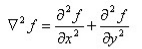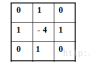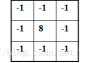2）LOG算法：

LOG算法步骤如下：1、 取样得到的高斯低通滤波器对输入图像滤波。2、 计算第一步得到图像的拉普拉斯。3、 找到步骤2所得图像的零交叉。

3)  Canny算子：Canny算子把边缘检测问题转换为检测单位函数极大值的问题来考虑。他利用高斯模型，借助图像滤波的概念指出一个好的边缘检测算子应该具有3个指标：1.低失误率，既要少将真的边缘丢弃，也要少将非边缘判为边缘；2.高位置精度，检测出的边缘应在真正的边界上；3.单像素边缘，即对每个边缘有唯一的响应，得到的边界为单像素宽。

1. 信噪比准则：信噪比越大，提取的边缘质量越高。信噪比SNR定义为

2.  定位精度准则边缘定位的精度L定义如下

3. 单边缘响应准则：为了保证单边缘只有一个响应。检测算子的脉冲响应导数的零交叉点的平均距离D(f’)应满足

(1) 用高斯滤波器平滑图像；(2)用一阶偏导的有限差分来计算梯度的幅值和方向；(3)对梯度幅值进行非极大值抑制；（排除非边缘像素，仅仅保留细线条）(4)用双阈值算法进行检测和链接边缘。

Robert：边缘定位精度较高，对于陡峭边缘且噪声低的图像效果较好，但没有进行平滑处理，没有抑制噪声的能力。

sobel和prewitt：进行了平滑处理，对噪声具有一定抑制能力，但容易出现多像素宽度。精度不高，边缘较粗糙。

Laplacian：对噪声较为敏感，使噪声能力成分得到加强，容易丢失部分边缘方向信息，造成一些不连续的检测边缘，抗噪声能力较差。

log：抗噪声能力较强，但会造成一些尖锐的边缘无法检测到。

canny：最优化思想的边缘检测算子，同时采用高斯函数对图像进行平滑处理，但会造成将高频边缘平滑掉，造成边缘丢失，采用双阈值算法检测和连接边缘。

4.形态学：在特殊领域运算形式——结构元素（Sturcture Element），在每个像素位置上与二值图像对应的区域进行特定的逻辑运算。运算结构是输出图像的相应像素。运算效果取决于结构元素大小内容以及逻辑运算性质。形态学，即数学形态学(mathematical Morphology），是图像处理中应用最为广泛的技术之一，主要用于从图像中提取对表达和描绘区域形状有意义的图像分量，使后续的识别工作能够抓住目标对象最为本质〈最具区分能力－most discriminative）的形状特征，如边界和连通区域等。同时像细化、像素化和修剪毛刺等技术也常应用于图像的预处理和后处理中，成为图像增强技术的有力补充。

4.1、膨胀（dilate）：膨胀就是求局部最大值的操作。从数学角度来说，就是将图像与核进行卷积，计算核B覆盖区域的像素点的最大值，并把这个最大值赋值给参考点指定的元素。这样就会使图像中的高亮区域逐渐增长。模板和输入图像对应位置的元素只要有一个与的结果不为0，则结果不为0.给图像中的对象边界添加元素。用3x3的结构元素，扫描二值图像中的每一个像素，用结构元素与其覆盖的二值图像做与运算，如果都为0，则结果图像中值为0，否则为1。结果：输入图像中的前景对象扩大一圈。

4.2、腐蚀（erode）：腐蚀和膨胀是相反的操作，腐蚀是求局部最小值的操作。腐蚀操作会使图像中的高亮区逐渐减小。模板和输入图像中对应位置的元素相与的结果全不为0时，结果才为0。删除对象边界的某些像素。用3x3的结构元素，扫描二值图像的每一个像素，用结构元素与其覆盖的二值图像做与运算，如果都为1，则结果图像中值为1，否则为0.结果：前景对象减小一圈。

4.3、开运算：开运算是先腐蚀后膨胀。主要用于消除小物体，在纤细点处分离物体，并且在平滑较大物体的边界的同时不明显改变其面积，同时抑制比结构元小的亮细节。

4.4、闭运算：是先膨胀后腐蚀。用来填充物体内细小空洞、连接邻近物体、平滑其边界的同时并不明显改变其面积，同时抑制比结构元小的暗细节。

4.5、形态学梯度：就是将膨胀图和腐蚀图相减。对二值化图像进行这一操作可以将边缘突出来，可以使用形态学梯度来保留物体的边缘轮廓。

4.6、顶帽变换：就是用源图像减去开运算图像。因为开运算带来的结果是放大了裂缝或者局部低亮度的区域。因此，从原图中减去开运算后的图，得到的效果图突出了比原图轮廓周围区域更明亮的区域。顶帽一般用于校正不均匀光照的影响（补充：均匀光照在从背景中提取目标的处理中扮演核心的角色）。

4.7、黑帽变换：就是用闭运算减去源图像。黑帽运算后的效果图突出了比原图轮廓周围的区域更暗的区域。黑帽运算一般用来分离比邻近点暗一些的斑块。

4.8、为什么开运算可以去除目标外的孤立点？

4.9、为什么闭运算可以去除目标内的孔？

6、图像金字塔：是图像中多尺度表达的一种，最主要用于图像分割，是一种以多分辨率来解释图像的有效但概念简单的结构。一幅图像的金字塔式一系列以金字塔形状排列的，分辨率逐步降低且来源于同一张原始图的图像集合。其通过梯次向下采样获得，直到到达某个终止条件才停止采样。金字塔的底部是待处理图像的高分辨率表示，而顶部是低分辨率的近似。层级越高图像越小，分辨率越低。

通常有两种类型的图像金字塔，分别是：

1）数字金字塔(Gaussuan pyramin)-用来向下采样，主要的图像金字塔，主要用来向下采样图像

2）拉普拉斯金字塔(Laplaican pyramid)-用来从金字塔底层图像重建上层未采样的还原，在数字图像处理中也即是预测残差，可以对图像进行最大程度的还原，配合高斯金字塔一起使用，是从金字塔底层图像中向上采样，重建一个图像。

6.1.高斯金字塔

a.对图像向下采样

为了获得第G(i+1)的金字塔图像，采取如下方法：

(1)对图像G(i)进行高斯内核卷积

(2)将所有偶数列和偶数行去除

得到的图像即为G(i+1)的图像，很明显G(i+1)只有源图像的四分之一，通过对输入图像G(i)(原始图像)不停的迭代上述步骤就会得到整个金字塔，即向下采样会丢失图像的信息，缩小了图像

b.对图像的向上采样

(1)将图像在每个方向上扩大为原来的两倍，新增的行和列以0填充

(2)使用先前同样的内核(乘以4)与放大后的图像卷积，获得“新增像素”的近似值。

6.2.拉普拉斯金字塔

i层的拉普拉斯金字塔的数学定义为:

L(i) = G(i) -UP(G(i+1))&g

6.3.尺寸调整:resize()函数

resize()函数是OpenCV中专门用来调整图像大小的函数

此函数将源图像精确的转换为指定尺寸的目标图像。如果源图像中设置了ROI(Region Of Internet,感兴趣区域)，那么resize()函数会对源图像的ROI区域进行调整图像尺寸的操作，来输出到目标图像中。若目标中已经设置了ROI区域，不难理解resize()函数将会对源图像进行尺寸调整并填充到目标图像的ROI中。

7二值化

7.1全局二值化：一幅图像包括目标物体、背景还有噪声，要想从多值的数字图像中直接提取出目标物体，最常用的方法就是设定一个全局的阈值T，用T将图像的数据分成两部分：大于T的像素群和小于T的像素群。将大于T的像素群的像素值设定为白色（或者黑色），小于T的像素群的像素值设定为黑色（或者白色）。

7.2局部自适应二值化：局部二值化也有一个缺陷。存在于那个统一阈值的选定。这个阈值是没有经过合理的运算得来，一般是取该窗口的平局值。这就导致在每一个窗口内仍然出现的是全局二值化的缺陷。为了解决这个问题，就出现了局部自适应二值化方法。

8、霍夫变换：霍夫变换是图像处理中用来从图像中分离出具有某种相同特征的几何形状（通常，直线，圆等）的常用方法。经典的霍夫变换常用来检测直线，圆，椭圆等。它是利用图像全局特性而将边缘像素连接起来组成区域封闭边界的一种方法。在预先知道区域形状的条件下，利用霍夫变换可以方便地得到边界曲线而将不连续的边缘像素点连接起来。霍夫变换的主要优点是受噪声和曲线间断的影响小。利用霍夫变换还可以直接检测某些已知形状的目标。

9.重映射：把一个图像中一个位置的像素放置到另一个图片指定位置的过程.简单的说就是改变图片的位置（左，右，上，下，颠倒）。

10、仿射变换： 仿射变换（Affine Transformation）是空间直角坐标系的变换，从一个二维坐标变换到另一个二维坐标，仿射变换是一个线性变换，他保持了图像的“平行性”和“平直性”，即图像中原来的直线和平行线，变换后仍然保持原来的直线和平行线，仿射变换比较常用的特殊变换有平移(Translation)、缩放（Scale）、翻转（Flip）、旋转（Rotation）和剪切(Shear)。

11、图像匹配：目前图像匹配中，局部特征匹配占据了绝大部分，常用的局部特征匹配方法有Harris、SIFT、SURF、ORB等等，不同的特征点检测和匹配方法尤其独特的优势和不足；

1.  特征检测（feature detection）、特征提取（extraction）和匹配（matching） 这三步，可以看做是目标检测、图像配准和拼接等工作的非常重要的一步。

2.  特征检测、特征选择、特征提取、特征描述和特征匹配

特征检测： 根据用户的需求在图像中寻找满足定义的特征，包括角点、Blob点和边缘点。检测的结果：有或没有。

特征选择： 为了选择稳定和可靠的特征，在检测到的特征集合中，需要进一步约束，通过类似于非极大值抑制、对比度阈值约束等条件保留显著特征。选择的结果：特征子集。

特征提取： 特征选择确定稳定可靠的特征子集后，需要提取特征的位置（Location）、方向（Orientation）和尺度（Scale）信息。方向和尺度信息主要是为支持旋转和尺度变化。

特征描述： 结合特征（点）邻域信息，使用一定的描述规则来对特征区域进行量化并抽取能代表该特征的描述信息，为了后续的匹配，一般用特征向量（feature vector）表示。

特征匹配： 对提取到的特征，需要通过使用一定的方法来进一步判断对应的特征是否相同（或近似），对特征向量一般使用欧式距离或最邻近距离比（NNDR）进行判定，满足一定的条件约束，则认为两个特征相近，否则剔除。一般还会通过RANSAC进一步约束剔除误匹配点。

## 《数字图像处理》复习提纲

2019-06-08 12:59:38 weixin_37545024 阅读数 785
• ###### 车道线检测相关理论知识点

通过本课程学习，初学者可以熟练使用opencv4 API完成各种常见的图像分割、识别任务。 高级学习者也一定能有新的体会和收获。 本课程所讲的例子代码来自于实际开发项目，有较高的实用性。

8人学习 刘山
免费试看

1、数字图像与图像处理

2、数字图像处理的特点：

1、数字图像处理的目的

2、数字图像处理的主要内容

## 第2章 数字图像处理基础

1、采样

2、量化

3、采样与量化参数的选择

4、图像数字化设备

### 2.2 色度学基础与颜色模型

1、色度学基础

2、颜色模型

（1）RGB模型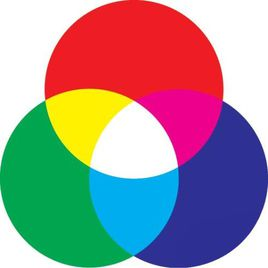（2）CIE XYZ模型
F=x•X+y•Y+z•Z, x=X/(X+Y+Z), y=Y/(X+Y+Z), z=Z/(X+Y+Z)
（3）Lab颜色模型

（4）HSI颜色模型

（5）CMY与CMYK颜色模型
（C，M，Y）=（1，1，1）-（R，G，B）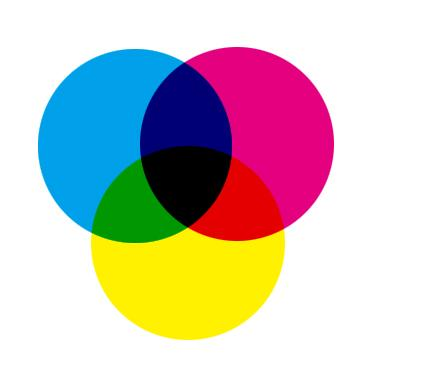（6）YUV电视信号彩色坐标系统
Y表示亮度信号，U、V表示色差
（7）YCbCr模型

3、颜色变换

1、位图

2、位图有关术语

### 2.4 图像文件格式

BMP图像文件格式：
（1）四部分组成：位图文件头、位图信息头、调色板、图像数据
（2）字节对齐，4byte整数倍
（3）上下倒置存放

### 2.5 OpenCV编程简介

OpenCV = Open Source Computer Vision Library
1、OpenCV简介 不考
2、OpenCV的安装与配置 不考
3、OpenCV的数据结构 不太可能考

4、读入、显示和存储图像

#include <iostream>
#include <opencv2/core/core.hpp>
#include <opencv2/highgui/highgui.hpp>
using namespace std;
using namespace cv;
int main(){
Mat image, result;
cout << "size: " << image.size().height() << " , " << image.size().width << endl;
if(!image.data){
cout << "read image file failed!" << endl;
}
namedWindow("Original Image");
imshow("Original Image", image);
flip(image, result,1);
namedWindow("Output Image");
imshow("Output Image", result);
imwrite("output.bmp", result);
waitKey(0);
return 0
}


5、操作图像像素
（1）at方法

void colorReduce(Mat& inputImage, Mat& outputImage, int div){
outputImage = inputImage.clone();
int rows = outputImage.rows;
int cols = outputImage.cols;
for(int i=0;i<rows;i++){
for(int j=0;j<cols;j++){
outputImage.at<Vec3b>(i,j) = outputImage.at<Vec3b>(i,j)/div*div+div/2;
outputImage.at<Vec3b>(i,j) = outputImage.at<Vec3b>(i,j)/div*div+div/2;
outputImage.at<Vec3b>(i,j) = outputImage.at<Vec3b>(i,j)/div*div+div/2;
}
}
}


（2）使用迭代器

void colorReduce(Mat& inputImage, Mat& outputImage, int div){
outputImage = inputImage.clone();
Mat_<Vec3b>::iterator it = inputImage.begin<Vec3b>();
Mat_<Vec3b>::iterator it = inputImage.end<Vec3b>();
Mat_<Vec3b> cimage = outputImage;
Mat_<Vec3b>::iterator itout = cimage.begin();
Mat_<Vec3b>::iterator itoutend = cimage.end();
for(; it != itend; it++,itout++){
(*itout)=(*it)/div*div+div/2;
(*itout)=(*it)/div*div+div/2;
(*itout)=(*it)/div*div+div/2;
}
}


（3）使用指针

void colorReduce(Mat& inputImage, Mat& outputImage, int div){
outputImage = inputImage.clone();
int rows = outputImage.rows;
int cols = outputImage.cols;
if(outputImage.isContinuous()){
cols = cols * rows;
rows = 1;
}
for(int i=0;i<rows;i++){
uchar * dataout = outputImage.ptr<uchar>(i);
for(int j=0;j<cols;j++){
dataout[j] = dataout[j]/div*div+div/2;
}
}
}


4、整行整列像素值的赋值

img.row(i).setTo(Scalar(255));
img.col(i).setTo(Scalar(255));


6、图形交互和媒体接口HighGui 不太可能考

## 第3章 图像增强

### 3.1 灰度变换

1、灰度线性变换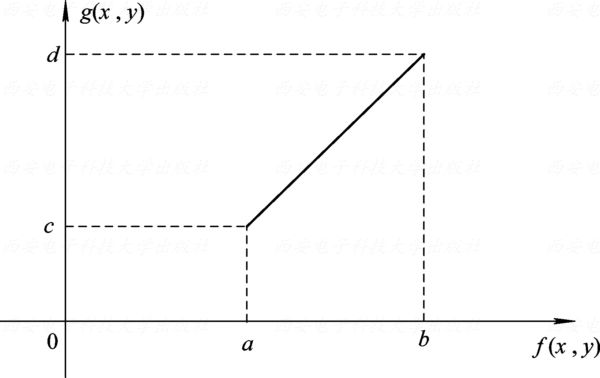2、分段线性变换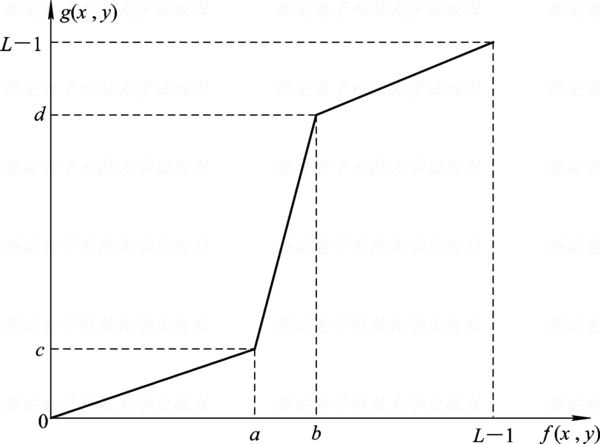3、非线性变换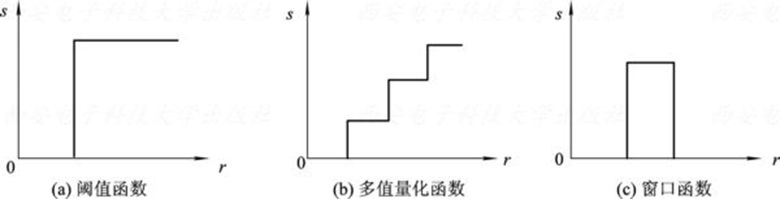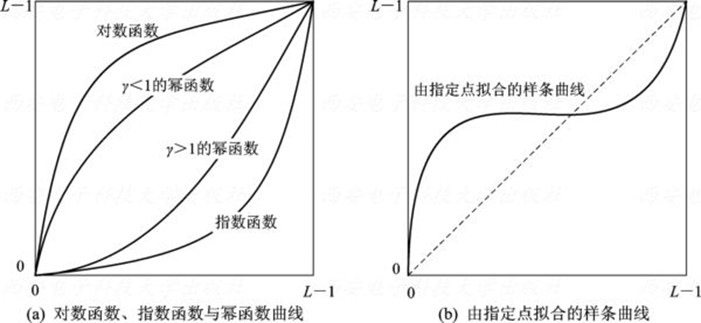### 3.2 直方图修正

1、直方图的基本概念

2、直方图的性质
（1）丢失位置信息，保留统计信息
（2）图像与灰度直方图是一对多的关系
（3）灰度直方图具有可加性
3、直方图的计算
（1）初始化：数组零值初始化
（2）统计：将量化灰度值映射为数组下标，遍历图像将对应数组元素的值++
（3）归一化：数组所有元素除以像素总数

4、直方图均衡化
（1）基本思想：把原始图像的直方图变换为均匀分布的形式，从而增加图像灰度的动态范围，以达到增强图像对比度的效果。经过均衡化处理的图像，其灰度级出现的概率相同，此时图像的熵最大，图像包含的信息量最大。
（2）推导
$s=T(r)$
s和r分别为灰度变换前后的灰度级，$T(r)$单调递增且有界[0,1]
$η=T(ξ)$，则有
$F_{η}(s) = P(η<s) = P(ξ<r) = \int_{-\infty}^{r}P_{r}(x)dx$

$p_{s}(s)=[p_{r}(r)•\frac{\mathrm{d} r}{\mathrm{d} s}]_{r=T^{-1}(s)}$

$p_s(s)=1$

$\mathrm{d} s=p_r(r)\mathrm{d}r$

$T(r)=s=\int_0^rp_r(ω)\mathrm{d}ω$

（3）数字图像的直方图均衡化

k rk nk pr=T(rk) sk=int[(L-1)sk+0.5] s rk->sk ps(sk)
0 0 790 0.19 0.19 0.19→1 0→1 -
1 1 1023 0.25 0.44 0.144→3 1→3 0.19
2 2 850 0.21 0.65 0.65→5 2→5 -
3 3 656 0.16 0.81 0.81→6 3→6 0.25
4 4 329 0.08 0.89 0.89→6 4→6 -
5 5 245 0.06 0.95 0.95→7 5→7 0.21
6 6 122 0.03 0.98 0.98→7 6→7 0.24
7 7 81 0.02 1 1→7 7→7 0.11

5、直方图规定化

rk,zl pr(rk) pz(zl) sk=T(rk) vl=G(zl) 单映射rk→zl 单映射ps(sk) 组映射rk→zl 组映射ps(sk)
0 0.19 - 0.19 - r0→r3 0 r0→r3 0
1 0.25 - 0.44 - r1→r3 0 r1→r5 0
2 0.21 - 0.65 - r2→r5 0 r2→r5 0
3 0.16 0.2 0.81 0.2 r3→r5 0.44 r3→r7 0.19
4 0.08 - 0.89 - r4→r7 0 r4→r7 0
5 0.06 0.5 0.95 0.7 r5→r7 0.37 r5→r7 0.46
6 0.03 - 0.98 - r6→r7 0 r6→r7 0
7 0.02 0.3 1 1 r7→r7 0.19 r7→r7 0.35

### 3.3 图像平滑

1、图像噪声
（1）噪声分类

（2）特点

2、模板卷积

3、邻域平均
Box模板：
$\frac{1}{9}\begin{bmatrix} 1 & 1 & 1 \\ 1 & 1* & 1 \\ 1 & 1 & 1 \end{bmatrix}$

$\frac{1}{16}\begin{bmatrix} 1 & 2 & 1 \\ 2 & 4* & 2 \\ 1 & 2 & 1 \end{bmatrix}$

4、中值滤波

（1）不影响阶跃信号、斜坡信号、连续个数少于窗口长度一半的脉冲受到抑制，三角函数顶部变平。
（2）中值滤波的输出与输入噪声的密度分布有关
（3）中值滤波频谱特性起伏不大，可以认为中值滤波之后，信号频谱基本不变

5、图像平均

### 3.4 图像锐化

1、微分法

（1）梯度算子

$\mathrm{▽}\mathbf{f}(x,y)=\begin{bmatrix}\frac{\partial f}{\partial x} & \frac{\partial f}{\partial y}\end{bmatrix}^T$

$\mathrm{▽} f(x,y)=|\mathrm{▽}\mathbf{f}(x,y)|=(G_x^2+G_y^2)^{\frac 1 2}$

$φ(x,y)=arctan(\frac {G_y} {G_x})$

$\mathrm{▽} f(x,y) \approx |f(x+1,y)-f(x,y)|+|f(x,y+1)-f(x,y)|$
Robert交叉算子
$\mathrm{▽} f(x,y) \approx |f(x+1,y+1)-f(x,y)|+|f(x,y+1)-f(x+1,y)|$

$\left \{\begin{matrix} G_x=\frac{f(x+1,y)-f(x-1,y)}{2} \\ \\ G_y=\frac{f(x,y+1)-f(x,y-1)}{2} \end{matrix}\right.$

Roberts $\begin{bmatrix}0* & -1 \\ 1 & 0\end{bmatrix}$ $\begin{bmatrix}-1* & 0 \\ 0 & 1\end{bmatrix}$ 各向同性；对噪声敏感；模板尺寸为偶数，中心位置不明显
Prewitt $\begin{bmatrix}-1 & 0 & 1 \\ -1 & 0* & 1 \\ -1 & 0 & 1 \end{bmatrix}$ $\begin{bmatrix}-1 & -1 & -1 \\ 0 & 0* & 0 \\ 1 & 1 & 1\end{bmatrix}$ 引入了平均因素，对噪声有抑制作用；操作简便
Sobel $\begin{bmatrix}-1 & 0 & 1 \\ -2 & 0* & 2 \\ -1 & 0 & 1 \end{bmatrix}$ $\begin{bmatrix}-1 & -2 & -1 \\ 0 & 0* & 0 \\ 1 & 2 & 1\end{bmatrix}$ 引入了平均因素，增强了最近像素的影响，噪声抑制效果比Prewitt要好
Krisch $\begin{bmatrix}-3 & -3 & 5 \\ -3 & 0* & 5 \\ -3 & -3 & 5 \end{bmatrix}$ $\begin{bmatrix}-3 & -3 & -3 \\ -3 & 0* & -3 \\ 5 & 5 & 5\end{bmatrix}$ 噪声抑制效果要好；需求出8个方向的响应（这里只给出两个方向的模板）
Isotropic Sobel $\begin{bmatrix}-1 & 0 & 1 \\ -\sqrt 2 & 0* & \sqrt 2 \\ -1 & 0 & 1 \end{bmatrix}$ $\begin{bmatrix}-1 & -\sqrt 2 & -1 \\ 0 & 0* & 0 \\ 1 & \sqrt 2 & 1\end{bmatrix}$ 权值反比于邻点与中心点的距离，检沿不同方向边缘时梯度幅度一致，即具有各向同性

（2）拉普拉斯算子

$\Delta f=\nabla ^2f=\frac{\partial ^2f}{\partial ^2x}+\frac{\partial ^2f}{\partial ^2y}$

$\nabla ^2f \approx f(x+1,y)+f(x-1,y)+f(x,y+1)+f(x,y-1)-4f(x,y)$

$\begin{bmatrix} 0 & -1 & 0 \\ -1 & 4* & -1 \\ 0 & -1 & 0 \end{bmatrix} \begin{bmatrix} -1 & -1 & -1 \\ -1 & 8* & -1 \\ -1 & -1 & -1 \end{bmatrix} \begin{bmatrix} 1 & -2 & 1 \\ -2 & 4* & -2 \\ 1 & -2 & 1 \end{bmatrix}$

（1）微分模板的权系数之和为0，使得灰度平坦区的响应为0。平滑模板的权系数都为正，和为1，这使得灰度平坦区的输出与输入相同。
（2）一阶微分模板在对比度较大的点产生较高的响应，二阶微分模板在对比度大的点产生零交叉。一阶微分一般产生更粗的边缘，二阶微分则产生更细的边缘。相对一阶微分而言，二阶微分对细线、孤立的点等小细节有更强的响应。
（3）平滑模板的平滑或去噪程度与模板的大小成正比，跳变边缘的模糊程度与模板的大小成正比。
2、非锐化滤波

$g(x,y)=f(x,y)-f_s(x,y)$

3、高频增强滤波

$f_{hb}(x,y)=Af(x,y)+Kg(x,y)$
$f_{hb}(x,y)$表示输出图像，$g(x,y)$$f(x,y)$的一个高通图像，A>=0，0<=K<=1。K在0.2~0.7之间取值时，高频增强滤波的效果最好；A足够大时，图像锐化作用削弱，输出图像接近原始图像整数倍；特别地，当A=1，K=1时为常用的拉普拉斯高频增强模板。

$\begin{bmatrix} 0 & -1 & 0 \\ -1 & 5* & -1 \\ 0 & -1 & 0 \end{bmatrix} \begin{bmatrix} -1 & -1 & -1 \\ -1 & 9* & -1 \\ -1 & -1 & -1 \end{bmatrix} \begin{bmatrix} 1 & -2 & 1 \\ -2 & 5* & -2 \\ 1 & -2 & 1 \end{bmatrix}$

### 3.5 伪彩色处理

1、灰度分层法：类似于等高线、卫星云图、天气预报图等
2、灰度变换法：红外热成像仪等
3、频域滤波法：在频率域进行伪彩色处理

### 3.6 同态滤波

$f(x,y)=i(x,y)r(x,y)$

$\mathrm{ln}f(x,y)=\mathrm{ln}i(x,y)+\mathrm{ln}r(x,y)$

$F_f(u,v)=F_i(u,v)+F_r(u,v)$

$H(u,v)=(\gamma _H-\gamma _L)[1- e^{-c\frac{D^2(u,v)}{D_0^2}}]+\gamma _L$
$\gamma _H$$\gamma _L$用于控制滤波强度，c用于控制滤波器斜面的锐化程度，$D^2(u,v)$为频谱上点（u，v)到频谱中心的距离，$D_0^2$为截止半径。

$g(x,y)=exp\{F^{-1}[F_f(u,v)H(u,v)]\}$

## 第4章 图像的几何变换

### 4.1 几何变换基础

1、概述
2、齐次坐标

3、二维图像几何变换矩阵
$P=TP_0 \\ P_0=\begin{bmatrix}x_1 & x_2 & ... & x_n \\ y_1 & y_2 & ... & y_n \\ 1 & 1 & ... & 1\end{bmatrix} \\ \\ P_=\begin{bmatrix}Hx_1' & Hx_2' & ... & Hx_n' \\ Hy_1' & Hy_2' & ... & Hy_n' \\ H & H & ... & H\end{bmatrix} \\ T=\begin{bmatrix}a & b & p \\ c & d & q \\ 0 & 0 & 1\end{bmatrix}$

### 4.2 图像比例缩放

1、图像比例放缩变换

（1）图像的比例缩小
$I(x,y)=F(int(cx),int(cy)), c=\frac 1 k$
（2）图像的非比例缩小
$I(x,y)=F(int(c_1x),int(c_2y)), c_1=\frac 1 {k_1}, c_2=\frac 1 {k_2}$
（3）图像的比例放大

$g(x,y)=(1-q)[(1-p)f([x],[y]+1)+pf([x]+1,[y])]+q[(1-p)f([x],[y]+1)+pf([x]+1,[y]+1)]$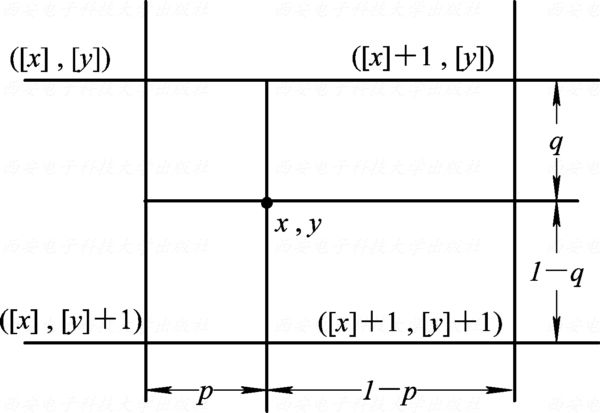2、比例缩放的实现 （略）

1、图像的旋转变换

2、图像旋转的实现（略）

### 4.6 图像复合变换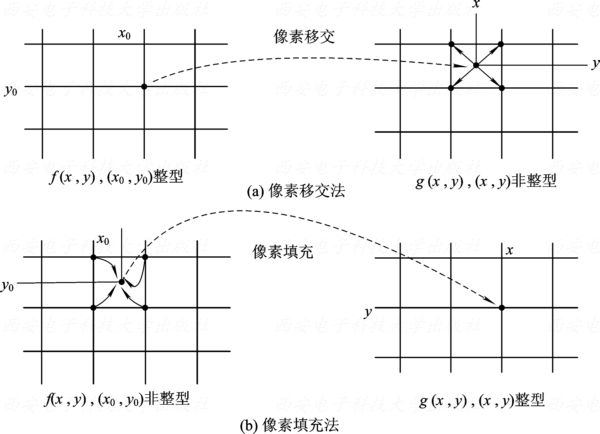1、透视变换

2、其他变换

（1）非矩形像素坐标的转换

（2）图像错切

（3）图像卷绕

### 4.8 几何畸变的矫正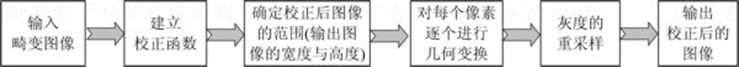（1）利用控制点对图像数据建立一个模拟几何畸变的数学模型，以建立畸变图像空间与标准空间的对应关系
（2）用这种对应关系把畸变空间中的全部元素变换到标准空间中去。

（1）直接成图法

$(x,y)=\boldsymbol f(u,v)$
（2）间接成图法（重采样成图法）

$(u,v)=\boldsymbol f^{-1}(x,y)$

$\left \{ \begin{matrix} u=g_u(x,y)=\sum_{i=0}^{n}\sum_{j=0}^{n-i}a_{ij}x^iy^j \\ \\ v=g_v(x,y)=\sum_{i=0}^{n}\sum_{j=0}^{n-i}b_{ij}x^iy^j \end{matrix} \right.$

$\left \{ \begin{matrix} \varepsilon_u=\sum_{k=1}^K(u_k-\sum_{i=0}^{n}\sum_{j=0}^{n-i}a_{ij}x_k^iy_k^j)^2 \\ \\ \varepsilon_v=\sum_{k=1}^K(v_k-\sum_{i=0}^{n}\sum_{j=0}^{n-i}b_{ij}x_k^iy_k^j)^2 \end{matrix} \right.$

## 第5章 频域处理

### 5.1 频域与频域变换

$f(x)\begin{matrix}正变换 \\ \Leftrightarrow \\ 逆变换\end{matrix}A(\omega),\Phi(\omega) \rightarrow F(\omega)$
5.2 傅里叶变换
1、连续函数的傅里叶变换

2、离散傅里叶变换

$\mathscr{F}[f(x)]=F(u)=\sum_{x=0}^{N-1}f(x)e^{-j\frac {2\pi ux}{N}} \\ \mathscr{F}^{-1}[F(u)]=f(x)=\frac 1 N \sum_{u=0}^{N-1}F(u)e^{j\frac {2\pi ux}{N}} \\ \mathscr{F}[f(x)]=F(u)=\sum_{x=0}^{N-1}f(x)[cos(\frac {2\pi} Nux)-j\cdot sin(\frac {2\pi} Nux)] \\ \mathscr{F}^{-1}[F(u)]=f(x)=\frac 1 N \sum_{u=0}^{N-1}F(u)[cos(\frac {2\pi} Nux)+j\cdot sin(\frac {2\pi} Nux)] \\$

$\mathscr{F}[f(x,y)]=F(u,v)=\sum_{x=0}^{M-1}\sum_{y=0}^{N-1}f(x,y)e^{-j2\pi (\frac {ux} M+\frac {vy} N)} \\ \mathscr{F}^{-1}[f(x,y)]=F(u,v)=\frac 1 {MN}\sum_{u=0}^{M-1}\sum_{v=0}^{N-1}F(u,v)e^{j2\pi (\frac {ux} M+\frac {vy} N)}$
3、离散傅里叶变换的性质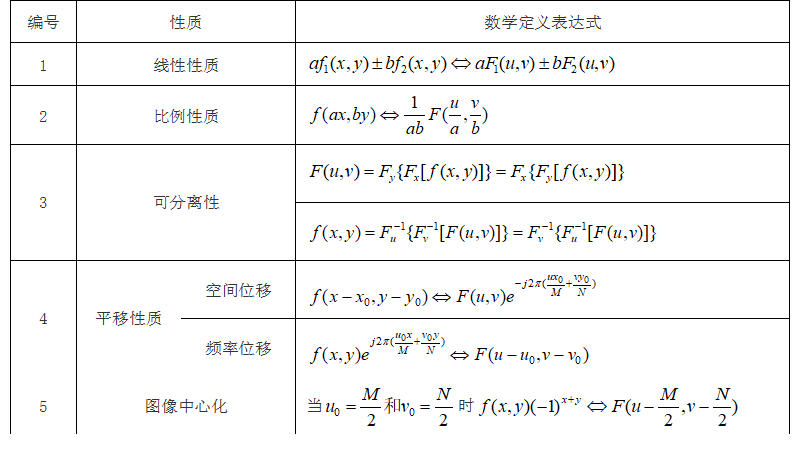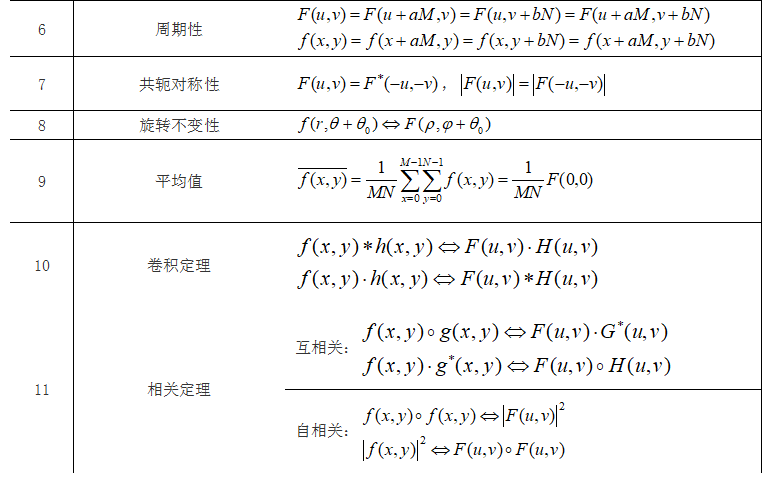4、离散傅里叶变换的openCV 不考

### 5.3 频域变换的一般表达式

1、可分离变换

$F(u,v)=\sum_{x=0}^{M-1}\sum_{y=0}^{N-1}f(x,y)\cdot g(x,y,u,v) \\ f(x,y)=\sum_{u=0}^{M-1}\sum_{v=0}^{N-1}F(u,v)\cdot h(x,y,u,v)$

$g(x,y,u,v)=g_1(x,u)\cdot g_2(y,v) \\ h(x,y,u,v)=h_1(x,u)\cdot h_2(y,v)$

2、图像变换的矩阵表示
$\boldsymbol {F=PfQ} \\ \boldsymbol {f=P^{-1}FQ^{-1}} \\ P(u,x)=g_1(x,u)=e^{-j\frac {2\pi ux} M} \\ Q(y,v)=g_2(y,v)=e^{-j\frac {2\pi vy} N}$

### 5.4 离散余弦变换（DCT）

1、一维离散余弦变换
DCT变换核定义：
$\begin{matrix} g(x,u)=C(u)\sqrt {\frac 2 N cos \frac {(2x+1)u\pi} {2N}} \\ \\ C(u)=\left \{ \begin{matrix} \frac 1 {\sqrt 2}, u=0 \\ \\ 1,else\end{matrix}\right. \end{matrix}$

$F(u)=\sum_{x=0}^{N-1}f(x)g(x,u) \\ \boldsymbol {F=Gf}(矩阵形式，G(u,x)=g(x,u)）$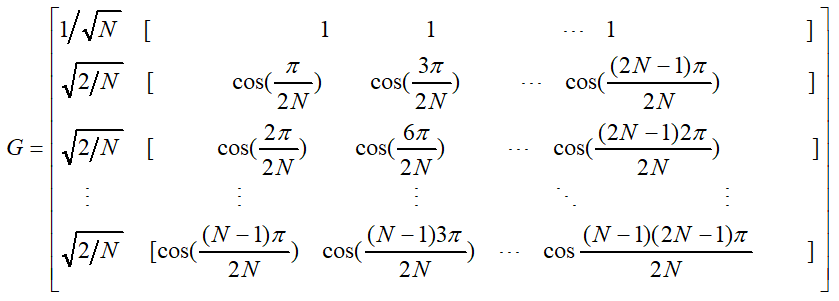DCT逆变换IDCT：
$h(x,u)=g(x,u)=C(u)\sqrt {\frac 2 N cos \frac {(2x+1)u\pi} {2N}} \\ f(x)=\sum_{u=0}^{N-1}F(u)h(x,u)$
2、二维离散余弦变换
2D-DCT：
$g_1(x,u)= \sqrt {\frac 2 M} C(u) cos \frac {(2x+1)u\pi} {2M} \\ g_2(y,v)= \sqrt {\frac 2 N} C(v) cos \frac {(2x+1)v\pi} {2N} \\ g(x,y,u,v)=g_1(x,u)g_2(y,v) \\ F(u,v)=\sum_{x=0}^{M-1}\sum_{y=0}^{N-1}f(x,y)g(x,y,u,v)$
2D-IDCT：
$h_1(x,u)=g_1(x,u) \\ h_2(y,v)=g_2(y,v) \\ h(x,y,u,v)=h_1(x,u)\cdot h_2(y,v) \\ f(x,y)\sum_{u=0}^{M-1}\sum_{v=0}^{N-1}F(u,v)h(x,y,u,v)$

### 5.5 频域中图像处理的实现

1、理解数字图像的频谱图

2、频域图像处理步骤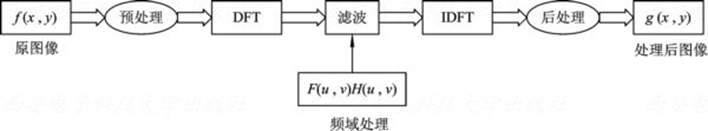3、频域滤波

## 第6章 数学形态学处理

1、数学形态学

2、基本符号和术语

### 6.2 二值形态学

1、腐蚀
$X\ominus S=\{x\in X |S+x\subseteq X\}$

2、膨胀
$X\oplus S=\bigcup\{X+s|s\in S\}=\bigcup\{S+x|x\in X\}=\{x|(S^v+x) \cap X\neq \varnothing\}$

$X\ominus(Y\cap Z)=(X\ominus Y)\cap(X\ominus Z) \\ X\oplus(Y\cap Z)=(X\oplus Y)\cap(X\oplus Z) \\ (X\cap Y)\ominus Z\supset(X\ominus Z)\cap (Y\ominus Z) \\ (X\cap Y)\oplus Z=(X\oplus Z)\cap (Y\oplus Z) \\ (X\cup Y)\ominus Z=(X\ominus Z)\cup (Y\ominus Z) \\ (X\cup Y)\oplus Z\subseteq(X\oplus Z)\cup (Y\oplus Z)$
3、开闭运算

（1）对偶性：$(X^C○S)^C=X\cdot S; (X^C\cdot S)=X○S$
（2）扩展性：$X○S\subseteq X \subseteq X\cdot S$

（3）单调性：$X\subseteq Y \Rightarrow X\cdot S\subseteq Y\cdot S, X○ S\subseteq Y○ S$
$Y\subseteq Z\wedge Z\cdot Y=Z \Rightarrow X\cdot Y\subseteq X \cdot Z$
（4）平移不变性：$(X+h)\cdot S=(X\cdot S)+h$
$(X+h)○ S=(X○ S)+h$
$X\cdot (S+h)=X\cdot S$
$X○ (S+h)=X○ S$
（5）等幂性：$(X\cdot S)\cdot S = X\cdot S,(X○ S)○ S = X○ S$
（6）开闭运算与集合的关系：
$(\bigcup_{i=0}^{n}X_i)○S\supseteq \bigcup_{i=0}^{n}(X_i○S)$
$(\bigcap_{i=0}^{n}X_i)○S\subseteq \bigcap_{i=0}^{n}(X_i○S)$
$(\bigcup_{i=0}^{n}X_i)\cdot S\supseteq \bigcup_{i=0}^{n}(X_i\cdot S)$
$(\bigcap_{i=0}^{n}X_i)\cdot S\subseteq \bigcap_{i=0}^{n}(X_i\cdot S)$
4、击中击不中变换
$X\odot S=\{x|S_1+x\subseteq X \wedge S_2+x\subseteq X^C\}=(X\ominus S_1)\cap(X^C\ominus S_2)$

### 6.3 灰值形态学

1、灰值腐蚀
$(f\ominus b)(s,t)=min\{f(s+x,t+y)-b(x,y)|s+x,t+y\in D_f \wedge x,y \in D_b\}$

2、灰值膨胀
$(f\oplus b)(s,t)=max\{f(s-x,t-y)+b(x,y)|s-x,t-y\in D_f \wedge x,y \in D_b\}$

$(f\oplus b)^C=f^C \ominus b^V$
$(f\ominus b)^C=f^C \oplus b^V$
3、灰值开闭运算

### 6.4 形态学的应用

1、形态学滤波

2、骨架提取

（1）基于腐蚀和开运算的细化

$S_k(X)=\bigcup\{S_k(X)|k=0,1,...,n\}$

$S_k(X)=(X\ominus kB)-[(X\ominus kB)○ B] \\ 递推公式：X\ominus kB=(X\ominus (k-1)B)\ominus B$

$S(X)=\bigcup\{(X\ominus kB)-[(X\ominus kB)○B]|k=0,1,2,...,n\}$

（2）快速形态学细化算法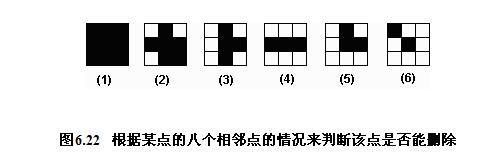（1）（2）（4）（6）都不能删除，（2）（4）可以删除

## 第7章 图像分割

### 7.1 阈值分割

1、概述

（1）种类：全局阈值、局部阈值、动态阈值（自适应阈值）
（2）阈值分割的要素：$T=T[x,y,f(x,y),p(x,y)]$, f(x,y)表示灰度值，p(x,y)表示某种局部性质。
2、全局阈值

（1）极小点阈值法：取直方图谷底位置作为阈值
（2）迭代阈值法：初始阈值$T_1$→像素划分为$G_1$$G_2$→计算平均灰度$\mu_1$$\mu_2$→计算新的阈值$T_2=\frac {\mu_1+\mu_2} 2$→迭代→终止条件$|T_2-T_1| <T_0$
（3）最优阈值法：选择一个阈值使得总的分类误差概率降到最低。
$p(z)$为灰度概率密度函数，$p_1(z)$$p_2(z)$分别为背景和目标灰度条件概率密度函数，$P_1$$P_2$为背景和目标像素出现的概率，那么由全概率公式得：
$p(z)=P_1p_1(z)+P_2p_2(z)$

$E_1(T)=\int_{-\infty}^Tp_1(z)\mathrm{d}z$

$E_2(T)=\int_T^{+\infty}p_2(z)\mathrm{d}z$

$E(T)=P_2E_1(T)+P_1E_2(T)$

$T=\frac {\mu_1+\mu_2} 2+\frac {\sigma^2} {\mu_1-\mu_2}ln(\frac{P_2}{P_1})$
（4）Otsu方法：使阈值分割后各个像素类的类间方差最大。

（5）p参数法

3、局部阈值
（1）直方图变换法

（2）散射图

4、动态阈值

1、区域生长

2、区域分裂与合并

### 7.3 边缘检测

1、微分算子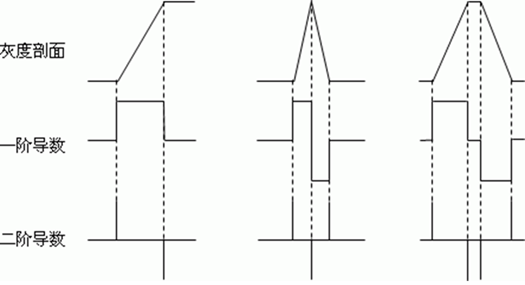（1）梯度算子：
Krisch、Prewitt，均可沿8个方向
（2）高斯-拉普拉斯算子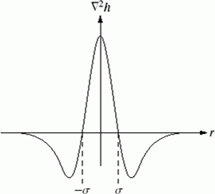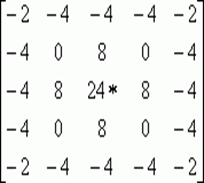（3）Canny边缘检测
Canny算子是一个非常有效的边界检测算子。
①首先对灰度图像进行高斯平滑。
②接着计算每个像素的梯度幅值和梯度方向。
③ 然后细化边缘。如果当前像素的梯度幅值不高于梯度方向上两个邻点的梯度幅值，则抑制该像素响应，这种方法称之为非最大抑制。
④ Canny算子使用两个幅值阈值，高阈值用于检测梯度幅值大的强边缘，低阈值用于检测梯度幅值较小的弱边缘。低阈值通常取为高阈值的一半。
⑤ 边缘细化后，就开始跟踪具有高幅值的轮廓。
⑥ 最后，从满足高阈值的边缘像素开始，顺序跟踪连续的轮廓段，把与强边缘相连的弱边缘连接起来。
2、边界连接

3、哈夫变换

（1）直线检测
y=ax+b→b=-ax+y→$\left \{\begin{matrix}b=-ax_i+y_i \\ b=-ax_j+y_j\end{matrix}\right.$（2）圆的检测：
$\left \{ \begin{matrix} a=x-rcos\theta \\ b=y-rsin\theta \end{matrix} \right.$
（3）任意曲线的检测：类似做法

1、区域标记

2、轮廓提取

3、轮廓追踪

### 7.5 分水岭分割

1、基本分水岭算法

2、Vincent-Soille算法

### 7.7 图像分割实例

（1）高斯滤波、中值滤波或开闭运算消噪
（2）消除非均匀光照：背景照度估计、灰值形态学、同态滤波
（2）区域分割

## 第8章 图像特征与理解

，全局特征用于描述图像或目标的颜色或形状等整体特征，局部特征指一些能够稳定出现并具有良好可区分性的特征。

### 8.1 图像的基本特征

1、几何特征
（1）位置和方向

（2）周长

（3）面积

①已知区域的行程编码，只需把值为1的行程长度相加，即为区域面积。
②若给定边界的某种表示，则相应连通区域的面积应为区域外边界包围的面积，减去它的内边界包围的面积（空的面积）
$y_k=y_0+\sum_{i=0}^k\Delta y_i \\ \Delta y_i=\left \{\begin{matrix}-1,\varepsilon _i = 1,2,3 \\ 0,\varepsilon _i = 0,4\\ 1,\varepsilon _i = 5,6,7 \\\end{matrix}\right. \\ \Delta x_i=\left \{\begin{matrix}-1,\varepsilon _i = 0,1,7 \\ 0,\varepsilon _i = 2,6\\ 1,\varepsilon _i = 3,4,5 \\\end{matrix}\right. \\ A=\sum_{i=1}^n(y_{i-1}\Delta x_i+a)$

（4）长轴和短轴

（5）距离

2、形状特征
（1）矩形度=物体面积/最小外接矩形面积

（2）圆形度：

3、球状性=内切圆半径/外接圆半径
4、偏心率=区域长轴/区域短轴
5、形状描述子
（1）边界链码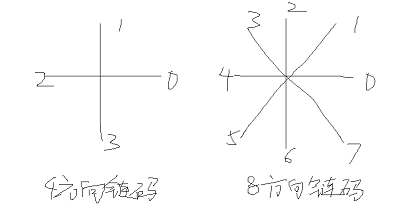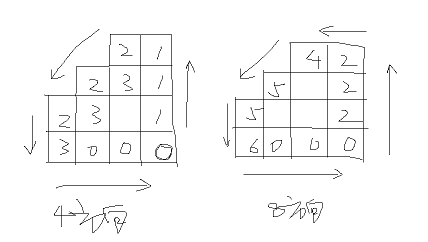（2）一阶差分链码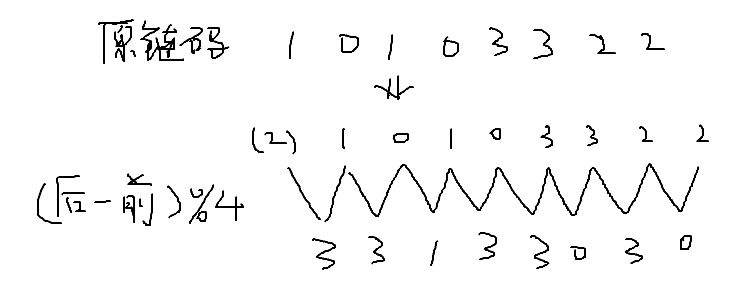（3）傅里叶描述子

### 8.2 角点特征

1、Moravec算法

$E(u,v)=\sum_{(x,y)\in W}(I(x+u,y+v)-I(x,y))^2$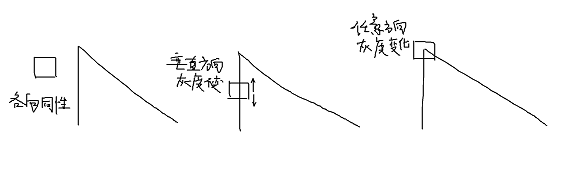2、Harris算法

$E(u,v)=\sum_{(x,y)\in W}w(x,y)(I(x+u,y+v)-I(x,y))^2 \\ w(x,y)=e^{-\frac {(x-x_0)^2+(y-y_0)^2} {2\sigma^2}} \\ 对I(x+u,y+v)进行泰勒展开：I(x+u,y+v)=I(x,y)+uI_x+vI_y \\ 代入上面的式子得:E(u,v)=[u,v]\boldsymbol M[u,v]^T \\ \boldsymbol M=\sum_{(x,y)\in W}w(x,y)\begin{bmatrix}I_x^2 & I_xI_y \\ I_xI_y & I_y^2 \end{bmatrix}=\begin{bmatrix}a & b \\ c & d \end{bmatrix}$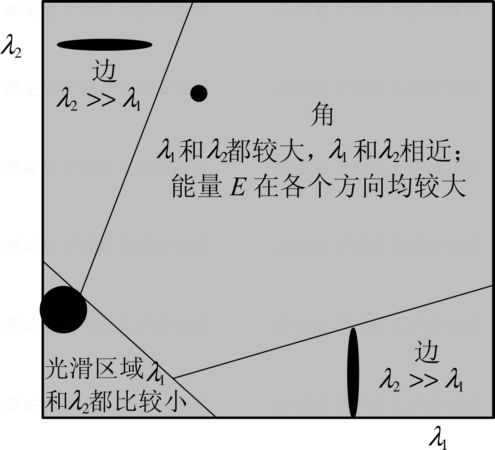3、SUSAN算法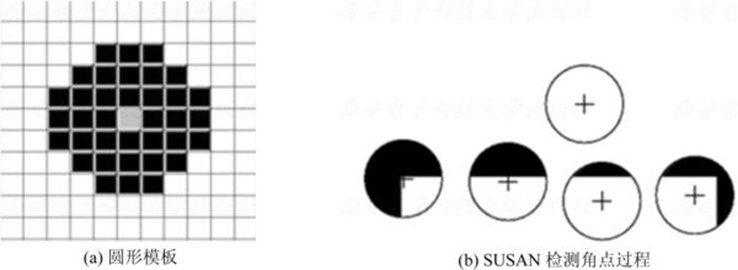## 第9章图像编码

### 9.1 图像编码概述

1、图像编码的基本原理和方法
（1）图像数据冗余：

（2）图像编码方法

①哈夫曼编码、香农-范诺编码、算术编码
②理论基础：平均码长等于信息熵时，总可设计出一种无损编码
③等长编码与变长编码
④变长编码定理：若一个离散无记忆信源的符号集具有r个码元，熵为H，则总可以找到一种无失真编码，构成单义可译码，使其平均码长L满足$\frac H {lbr}\le L \le \frac H {lbr}+1$

2、图像编码新技术（略）
3、图像编码评价

$L=\sum_{i=1}^{N}p_iL_i \\ C_R=\frac B L \\ \eta = \frac H L$

### 9.3 香农-范诺编码

1、基本方法：

2、二分法香农-范诺编码方法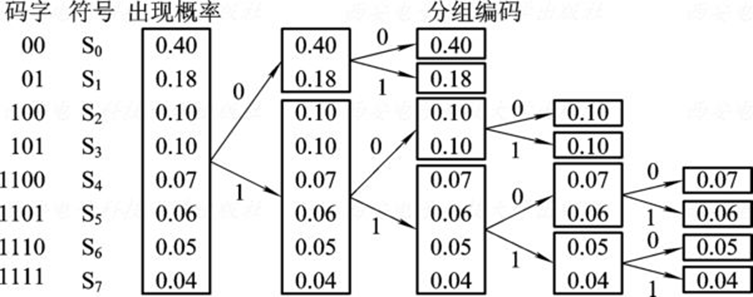### 9.4 算术编码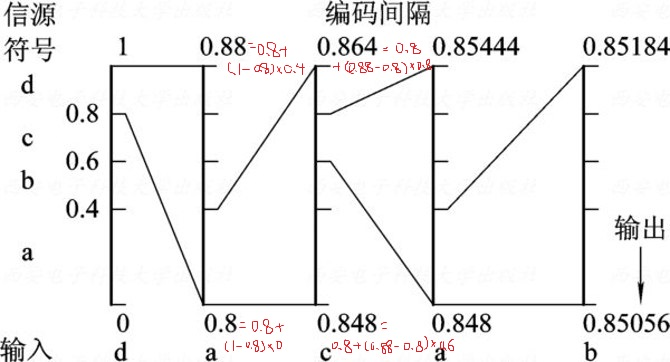### 9.5 行程编码

aabbbcddddd=2a3b1c5d

### 9.6 LZW编码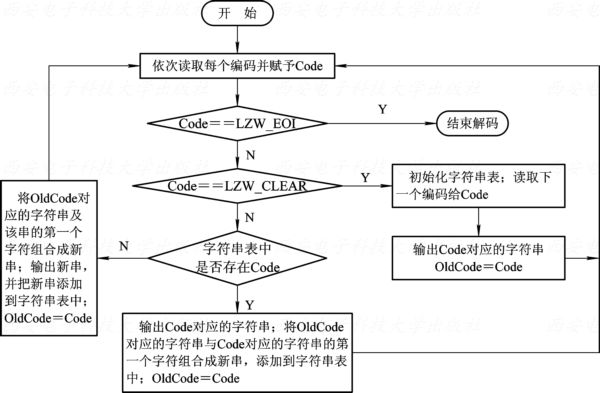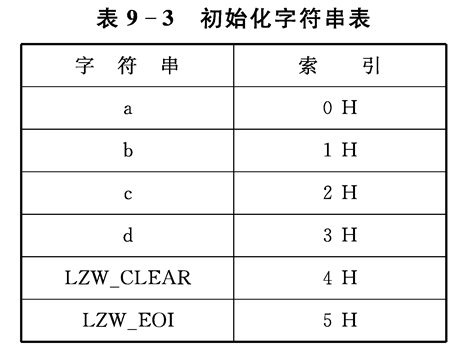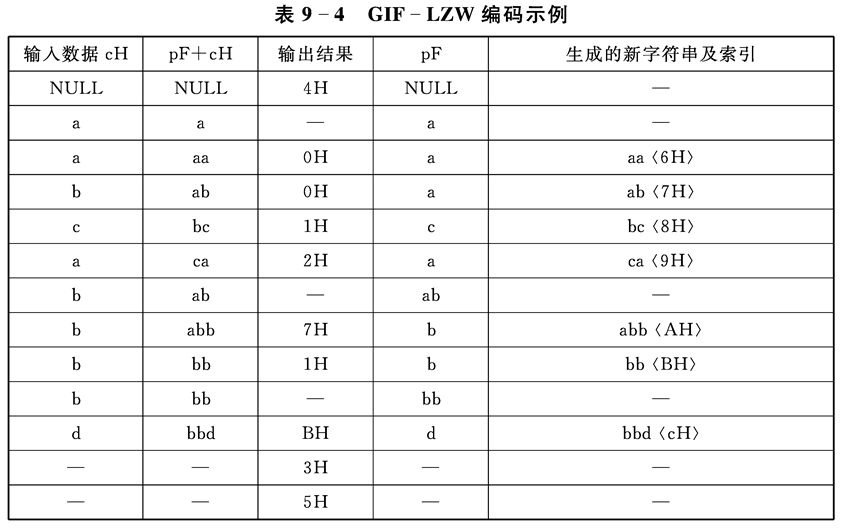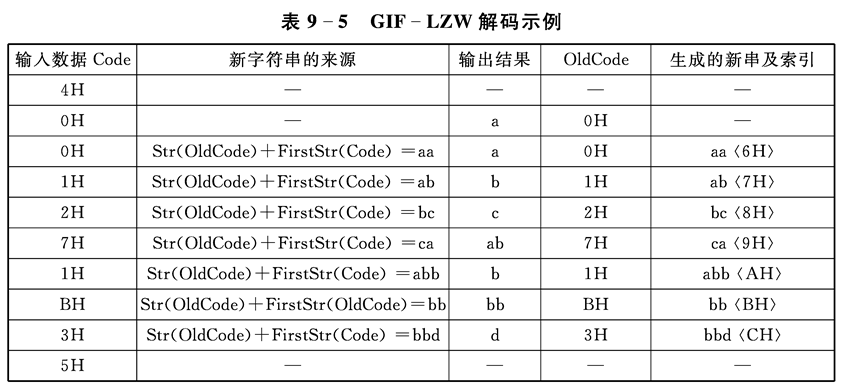## OpenCV Image Flip 图像翻转

2018-07-12 23:42:33 yc461515457 阅读数 1148
• ###### 车道线检测相关理论知识点

通过本课程学习，初学者可以熟练使用opencv4 API完成各种常见的图像分割、识别任务。 高级学习者也一定能有新的体会和收获。 本课程所讲的例子代码来自于实际开发项目，有较高的实用性。

8人学习 刘山
免费试看

# Flip

// C++:

void cv::flip(InputArray src, OutputArray dst, int flipCode )

# Python:

dst = cv.flip(src, flipCode[, dst])


## Parameters

src input array.
dst output array of the same size and type as src.
flipCode a flag to specify how to flip the array; 0 means flipping around the x-axis and positive value (for example, 1) means flipping around y-axis. Negative value (for example, -1) means flipping around both axes.